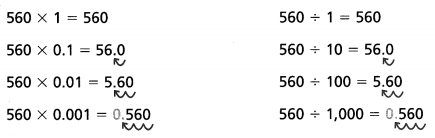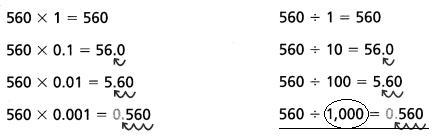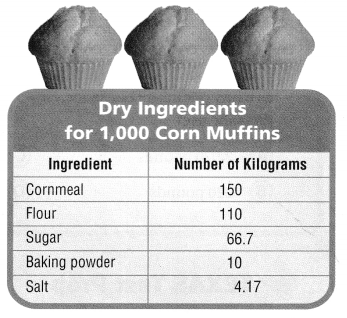# Texas Go Math Grade 5 Lesson 4.1 Answer Key Division Patterns with Decimals

Refer to our Texas Go Math Grade 5 Answer Key Pdf to score good marks in the exams. Test yourself by practicing the problems from Texas Go Math Grade 5 Lesson 4.1 Answer Key Division Patterns with Decimals.

## Texas Go Math Grade 5 Lesson 4.1 Answer Key Division Patterns with Decimals

Unlock the Problem

The Healthy Wheat Bakery uses 560 pounds of flour to make 1,000 loaves of bread. Each loaf contains the same amount of flour. How many pounds of flour are used in each loaf of bread?

You can use place value patterns to help you find quotients. Dividing by 10, 100, or 1,000 is the same as multiplying by 0.1, 0.01, or 0.001.

• Underline the sentence that tells you what you are trying to find.
• Circle the numbers you need to use.

Use place-value patterns

Divide. 560 ÷ 1,000
Look for a pattern in these products and quotients.So, __________ pound of flour is used in each loaf of bread.Explanation:
So, 0.560 pound of flour is used in each loaf of bread.

Question 1.
As you divide by 10,100, and 1,000, how does the position of the decimal point change in the quotients?

Move the decimal point to the left for as many places (steps) as there are zeros in the factor 10, 100, or 1000.
Explanation:

28 ÷ 10=2.8  — Move the decimal point one step to the left.
28÷ 100 = 0.28 –Move the decimal point two steps to the left. You need to write zeros in front of the number.
28 ÷ 1000 = 0.028 — Move the decimal point three steps to the left. You need to write zeros in front of the number.

Try This! Complete the pattern.

A.
32.6 ÷ 1 = __________
32.6 ÷ 10 = __________
32.6 ÷ 100 = __________
32.6 ÷ 1 = 32.6
32.6 ÷ 10 = 3.26
32.6 ÷ 100 =0.326
Explanation:
Move the decimal point to the left for as many places (steps) as there are zeros in the factor 10, 100, or 1000..

B.
150.2 ÷ 1 = __________
150.2 ÷ 10 = __________
150.2 ÷ 100 = _________
150.2 ÷ 1 = 150.2
150.2 ÷ 10 = 15.02
150.2 ÷ 100 = 1.502
Explanation:
Move the decimal point to the left for as many places (steps) as there are zeros in the factor 10, 100, or 1000.

Math Talk
Mathematical Processes

Explain how you can determine where to place the decimal point in the quotient 47.3 ÷ 100.
Explanation:
Move the decimal point two steps to the left. You need to write zeros in front of the number.

Example

Liang used 25.5 pounds of tomatoes to make a large batch of salsa. He used one-tenth as many pounds of onions as pounds of tomatoes. He used one-hundredth as many pounds of green peppers as pounds of tomatoes. How many pounds of each ingredient did Liang use?
Tomatoes: 25.5 pounds
Onions: 25.5 pounds ÷ _________
Think: 25.5 ÷ 1 =
25.5 ÷ 10 = ______

Green Peppers: 25.5 pounds ÷ _________
Think ________ ÷ 1 = _________
_________ ÷ 10 = _________
_________ ÷ 100 = _________
So, Liang used 25.5 pounds of tomatoes, _________ pounds of onions, and _________ pound of green peppers.
Tomatoes: 25.5 pounds
Onions: 25.5 pounds ÷ 10
Think: 25.5 ÷ 1 =25.5
25.5 ÷ 10 =2.55

Green Peppers: 25.5 pounds ÷ 100
Think 25.5 ÷ 1 = 25.5
25.5 ÷ 10 =2.55
25.5÷ 100 = 0.255
So, Liang used 25.5 pounds of tomatoes, 2.55 pounds of onions, and  0.255  pound of green peppers.
Explanation:
Move the decimal point two steps to the left. You need to write zeros in front of the number

Math Talk
Mathematical Processes

Explain what happens to the value of a number when you divide by 10, 100, or 1,000.
The decimal point will move to the left.
Explanation:
The place value changes. You need to write zeros in front of the number.

Share and Show

Complete the pattern.

Question 1.
456 ÷ 1 = 456
456 ÷ 10 = 45.6
456 ÷ 100 = 4.56
456 + 1000 = __________
Think: The dividend is being divided by 10, 100, and 1,000 so the decimal point will move to the _________ one place for each equation.
Explanation:
The dividend is being divided by 10, 100, and 1,000 so the decimal point will move to the  left one place for each equation.
Question 2.
1,225 ÷ 1 = ________
1,225 ÷ 10 = ________
1,225 ÷ 100 = ________
1,225 ÷ 1,000 = ______
1,225 ÷ 1 = 1225
1,225 ÷ 10 = 122.5
1,225 ÷ 100 =12.25
1,225 ÷ 1,000 =1.225
Explanation:
Move the decimal point to the left for as many places (steps) as there are zeros in the factor 10, 100, or 1000.

Question 3.
605 ÷ 1 = ________
605 ÷ 10 = ________
605 ÷ 100 = _____
605 ÷ 1,000 = ________
605 ÷ 1 =605
605 ÷ 10 = 60.5
605 ÷ 100 =6.05
605 ÷ 1,000 = 0.605
Explanation:
Move the decimal point to the left for as many places (steps) as there are zeros in the factor 10, 100, or 1000.

Question 4.
74.3 ÷ 1 = ________
74.3 ÷ 10 = ________
74.3 ÷ 100 = ________
74.3 ÷ 1 = 74.3
74.3 ÷ 10 = 7.43
74.3 ÷ 100 = 0.743
Explanation:
Move the decimal point to the left for as many places (steps) as there are zeros in the factor 10, 100, or 1000.

Problem Solving

H.O.T. Algebra Find the value of n.

Question 5.
268 ÷ n = 0.268
n = _____________
Explanation:
when 268 is divided by 1000

Question 6.
n ÷ 100 = 0.123
n = _____________
Explanation:
When 12.3 divided by 100

Question 7.
n ÷ 10 = 4.6
n = ______________
Explanation:
When 46 is divided 10

Question 8.
Write Math Explain how you know that the quotient 47.3 is equal to the product 47.3 × 0.1.
Explanation:
When 47.3 is divided with 10 the answer is 4.73
and the same number is multiplied with 0.1 the answer is 4.73

Problem Solving

Use the table to solve 9-11.Question 9.
Multi-Step About how much more cornmeal than flour does each muffin contain?
Explanation:
As cornmeal is 150 and flour is 110
150 – 110 = 40

Question 10.
H.O.T. If each muffin contains the same amount of sugar, how many kilograms of sugar, to the nearest thousandth, are in each corn muffin?Explanation:
If each muffin contains the same amount of sugar,
0.0667 kilograms of sugar, to the nearest thousandth, are in each corn muffin

Question 11.
H.O.T. Multi-Step The bakery decides to make only 100 corn muffins on Tuesday. How many kilograms of sugar will be needed?
Explanation:
The bakery decides to make only 100 corn muffins on Tuesday.
0.667 kilograms of sugar will be needed

Question 12.
Orange crayons are usually made in batches of 100. If it takes 22 pounds of wax to make 100 orange crayons, then how much wax will you need to make one orange crayon?
(A) 0.22 pound
(B) 22 pounds
(C) 2 pounds
(D) 0.022 pound
Explanation:
Orange crayons are usually made in batches of 100.
If it takes 22 pounds of wax to make 100 orange crayons,
0.22 pound of  wax will be need to make one orange crayon

Question 13.
Use Symbols A famous barbecue restaurant in Texas makes 1,000 pounds of barbecue pork each week. The owner has a catering order that requires 100 pounds of barbecue pork. The owner uses 44 pounds of sauce for every 1,000 pounds of barbecue pork. Which equation shows the amount of sauce needed for the catering order?
(A) 44 ÷ 1 = 44 pounds
(B) 44 ÷ 10 = 4.4 pounds
(C) 44 ÷ 100 = 0.44 pound
(D) 44 ÷ 1,000 = 0.044 pound
Explanation:
A famous barbecue restaurant in Texas makes 1,000 pounds of barbecue pork each week.
The owner uses 44 pounds of sauce for every 1,000 pounds of barbecue pork.
44 ÷ 1,000 = 0.044 pound
0.044 x 100 = 4.4
so, 44 ÷ 10 = 4.4 pounds

Question 14.
Multi-Step Loretta is trying to build the largest taco in the world. She uses 2,000 pounds of ground beef, one-tenth as many pounds of cheese, one-hundredth as many pounds of lettuce, and one-thousandth as many pounds of hot sauce. How many pounds of lettuce and cheese does she use?
(A) 2,020 pounds
(B) 220 pounds
(C) 2,200 pounds
(D) 20 pounds
Explanation:
Loretta is trying to build the largest taco in the world.
She uses 2,000 pounds of ground beef, one-tenth as many pounds of cheese, 2000 ÷ 10 = 200
one-hundredth as many pounds of lettuce, 2000 ÷ 100 = 20
and one-thousandth as many pounds of hot sauce.
220 pounds of lettuce and cheese  she use, 200 + 20 = 220

Texas Test Prep

Question 15.
Apply Ella used 37.2 pounds of apples to make applesauce. She used one-tenth as many pounds of sugar as pounds of apples. How many pounds of sugar did Ella use?
(A) 0.372 pound
(B) 3.72 pounds
(C) 372 pounds
(D) 0.0372 pound
Explanation:
Apply Ella used 37.2 pounds of apples to make applesauce.
She used one-tenth as many pounds of sugar as pounds of apples.
3.72 pounds of sugar used by Ella
37.2  ÷ 10 = 3.72

### Texas Go Math Grade 5 Lesson 4.1 Homework and Practice Answer Key

Find the value of n.

Question 1.
456 ÷ n = 4.56
n = _____________
Explanation:
When 456 is divided by 100

Question 2.
n ÷ 100 = 0.982
n = _____________
Explanation:
When 98.2 is divided by 100

Question 3.
n ÷ 10 = 32.7
n = ______________
Explanation:
When 327 is divided by 10

Question 4.
32 ÷ 1,000 = n
n = ______________
Explanation:
When 32 is divided by 1000

Question 5.
1,672 ÷ n= 1.672
n = _______________
Explanation:
When 1672 is divided by 1000

Question 6.
24 ÷ 100 = n
n = _______________
Explanation:
When 24 is divided by 100

Question 7.
9,871 ÷ n = 987.1
n = ______________
When 9871 is divided by 10

Question 8.
n ÷ 10 = 5.69
n = ______________
Explanation:
When 56.9 is divided by 10

Question 9.
n ÷ 1,000 = 0.823
n = _______________
Explanation:
When 823 is divided by 1000

Problem Solving

Question 10.
Mr. Baker drives 24.5 miles to his cousin’s house. He drives one-tenth as many miles to his nephew’s house. How many miles does Mr. Baker drive to his nephew’s house?
Explanation:
Mr. Baker drives 24.5 miles to his cousin’s house.
He drives one-tenth as many miles to his nephew’s house.
24.5÷10 =2.45
Mr. Baker drive to his nephew’s house

Question 11.
Elena sews sequins on a skirt for the dance recital. She sews 100 sequins end-to-end along the length of the skirt. The skirt is 50 centimeters long. What is the length of one sequin?
Explanation:
Elena sews sequins on a skirt for the dance recital.
She sews 100 sequins end-to-end along the length of the skirt.
The skirt is 50 centimeters long.
50 ÷1=50

Lesson Check

Question 12.
An online bookstore ships boxes with a copy of the new bestseller in each box. The total weight of the shipment is 1,200 pounds. If each box weighs 1.2 pounds, how many boxes are shipped?
(A) 10
(B) 10,000
(C) 1,000
(D) 100
Explanation:
An online bookstore ships boxes with a copy of the new bestseller in each box.
The total weight of the shipment is 1,200 pounds.
If each box weighs 1.2 pounds
1000 boxes are shipped

Question 13.
A car dealership uses 1,350 gallons of gasoline to fill the tanks of 100 of their compact cars. How much gas does each tank hold?
(A) 13.5 gallons
(B) 1.35 gallons
(C) 135 gallons
(D) 0.135 gallon
Explanation:
A car dealership uses 1,350 gallons of gasoline to fill the tanks of 100 of their compact cars.
1350 ÷ 100 = 13.50 pounds

Violet sells homemade granola at the Saturday market. Her recipe makes 10 large bags of granola or 100 snack-size bags. Use Violet’s recipe at the right for 14-16.Question 14.
Which equation shows the number of grams of almonds in one large bag?
(A) 750.2 ÷ 1 = 750.2 grams
(B) 750.2 ÷ 10 = 75.02 grams
(C) 750.2 ÷ 100 = 7.502 grams
(D) 750.2 ÷ 1,000 = 0.7502 gram
Explanation:
Her recipe makes 10 large bags of granola
for 10 large bags she uses 750.2 grams of almonds
750.2 ÷ 10 = 75.02

Question 15.
Multi-Step What is the total number of grams of oats and sunflower seeds in one large bag?
(A) 345.05 grams
(B) 750.5 grams
(C) 45.05 grams
(D) 120.07 grams
Explanation:
Her recipe makes 10 large bags of granola
For 10 large bags she uses 3000 grams of oats and 450.5 grams of sunflower seeds
3450.5 ÷ 10 = 345.05

Question 16.
Multi-Step How many grams of sunflower seeds are in two snack-size servings of granola?
(A) 4.505 grams
(B) 45.05 grams
(C) 91 grams
(D) 9.01 grams